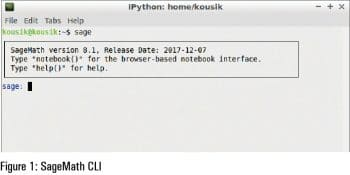# Introducing SageMath: A System for Algebraic and Geometrical Experimentation

0
3930There are numerous platforms, like MATLAB, Mathematica, Magma, Maple, etc, for studying mathematical principles, calculation and computation. There are also tools for drawing graphs. SageMath is another tool for your maths arsenal, from the world of open source.

SageMath is an open source (released under GPLv3) Python based, mathematics application that supports research and teaching in algebra, geometry, number theory, cryptography, numerical computation, and related subjects. The principle underlying SageMath is to provide an open source alternative to MATLAB, Mathematica, Magma, and Maple. It is more advanced than any of the graphing calculators. And as it is Python based, users learn Python in the process of using SageMath.

Important features

Sage includes a browser based notebook for the review and re-use of previous inputs and outputs, including graphics and text annotations. It is compatible with most of the common Web browsers like Firefox, Opera, Konqueror, Google Chrome and Safari. Notebooks can also be hosted on the server, and be accessed by HTTPS from the client system.

SageMath has a text based command-line interface that uses IPython. It supports parallel processing by using multi-core processors, multiple processors, or distributed computing. It also supports:

• Calculus by using Maxima and SymPy
• Numerical linear algebra by using the GSL, SciPy and NumPy
• Libraries of elementary and special mathematical functions
• 2D and 3D graphs of symbolic functions and numerical data
• Matrix manipulation, including sparse arrays
• Multi-variate statistics libraries, using R and SciPy
• A toolkit for adding user interfaces to calculations and applications
• Graph theory visualisation and analysis tools
• Libraries of number theory functions
• Support for complex numbers, arbitrary-precision arithmetic, and symbolic computation
• Technical word processing, including formula editing and embedding SageMath within LaTeX documents
• The Python standard library, including tools for connecting to SQL, HTTP, HTTPS, NNTP, IMAP, SSH, IRC, FTP and others.
• ▪ Interfaces to some third-party applications like Mathematica, Magma, R, and Maple.Installation

SageMath can be installed in any of the following OSs — Linux, macOS, Microsoft Windows, Solaris, Android and iOS. You can get it from http://www.sagemath.org/download.html.

SageMath is present in the Ubuntu repository. You can install it by using the following command:

`\$sudo apt-get install sagemath`

This will take time as the package is a bit heavy. There is one more thing you have to do – if you have the Anaconda (a Python/R data science and machine learning tool) path in your bashrc, then comment it out before starting.

Working with SageMath

To start in text mode, run the following command:

`\$sage`

Now SageMath is ready for you to work on. You can use Sage in a terminal. It also provides you a browser based notebook interface.

Type notebook() at the Sage prompt. It will ask you to set the admin password. After setting the password, the Sage notebook page will be opened automatically.

Basic operations

The basic arithmetic operations are:

```“four operations” a+b, a-b, a*b, a/b

power a^b or a**b

square root sqrt(a)

n-th root a^(1/n)```

The basic integer operations are:

```integer division a // b

remainder a % b

quotient and remainder divmod(a,b)

factorial n! factorial(n)

binomial coefficient n k binomial(n,k)```
```integer part floor(a)

absolute value, modulus abs(a)

elementary functions sin, cos```

2D or 3D graphs can also be plotted by Sage. To plot a 3D graph, you need to have Java configured in your system. The plot of a 2D graph is shown in Figure 4.

To get help, you have to type the following command:

```sage: keyword?

Here I run

sage: cot?```